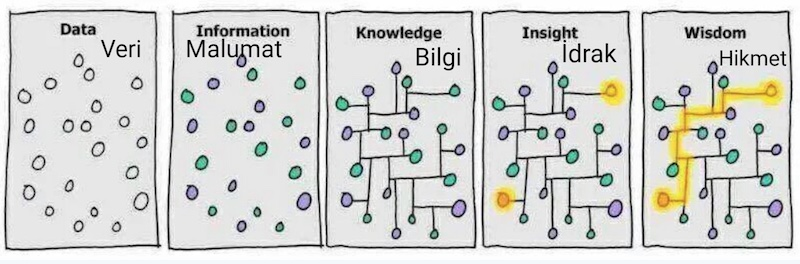December 13, 2018

## Summary of the course

• Keep your data in text files
• Read data from a text file
• Modify your data frame to simplify the analysis
• select rows and columns
• Calculate summary statistics and models
• Draw nice plots to show your results
• Write text explaining the results

## SummaryIt is hard to make predictions, especially about the future

We can try to predict based in the past

We know the midterm grades, the attendance to classes and quizzes, and the survey you filled at the beginning of the semester

## First data: official list from Aksis

head(aksis)
      Numara      Ad   Soyad before
1 0405140001 GÜLSELİ  KIRGIL   TRUE
2 0405140013   TUĞÇE     CAN   TRUE
3 0405140023    AYŞE   DALLI  FALSE
4 0405140028   BÜŞRA   KAYIN   TRUE
5 0405140051 FİKRİYE  ÖZKAYA   TRUE
6 0405140052   İLYAS BERKTAŞ  FALSE

## Second data: Class attendance

head(att)
      Numara att12 att21
1 0405140001     0     0
2 0405140013     3     9
3 0405140023    12    21
4 0405140028     1     3
5 0405140051     0     0
6 0405140052     9    15

## Combining two data frames

The two data frames have one column in common

cmb1 <- merge(aksis, att, by="Numara")
head(cmb1)
      Numara      Ad   Soyad before att12 att21
1 0405140001 GÜLSELİ  KIRGIL   TRUE     0     0
2 0405140013   TUĞÇE     CAN   TRUE     3     9
3 0405140023    AYŞE   DALLI  FALSE    12    21
4 0405140028   BÜŞRA   KAYIN   TRUE     1     3
5 0405140051 FİKRİYE  ÖZKAYA   TRUE     0     0
6 0405140052   İLYAS BERKTAŞ  FALSE     9    15

## Combine with Quizzes delivery

head(quiz)
      Numara nquiz
1 0405140001     0
2 0405140013     0
3 0405140023     0
4 0405140028     1
5 0405140051     0
6 0405140052     0
cmb1 <- merge(cmb1, quiz, by="Numara")

head(midterm)
      Numara grade
1 0405140013    64
2 0405140023    14
3 0405140028    23
4 0405140051     0
5 0405140052     0
6 0405140070    52
cmb1 <- merge(cmb1, midterm, by="Numara")

## Combine with Survey

head(sv, 1)
      Numara              english believe_professor
1 0405110024 I can speak fluently   mostly disagree
believe_internet            lazy         learner
1  mostly disagree mostly disagree mostly disagree
nocalendar believe_journal           chess
1 mostly disagree mostly disagree mostly disagree
improviser        hardwork       time_mgmt
1 mostly disagree mostly disagree mostly disagree
memory          genius       curiosity
1 mostly disagree mostly disagree mostly disagree
must_be_correct    hate_surveys     disciplined
1 mostly disagree mostly disagree mostly disagree
need_order    all_in_books    like_puzzles
1 mostly disagree mostly disagree mostly disagree
books week_study cmb_study scientist_any_other
1     9          6         1     mostly disagree
scientist_robots scientist_not_creative
1  mostly disagree        mostly disagree
scientist_like_artists
1        mostly disagree
cmb1 <- merge(cmb1, sv, by="Numara", all.x=TRUE)

## Summary

dim(cmb1)
 63 35
summary(cmb1[c(4,5,7)])
   before            att12            nquiz
Mode :logical   Min.   : 0.000   Min.   :0.000
FALSE:55        1st Qu.: 8.000   1st Qu.:1.000
TRUE :8         Median :10.000   Median :2.000
Mean   : 9.111   Mean   :2.413
3rd Qu.:11.000   3rd Qu.:4.000
Max.   :12.000   Max.   :6.000  

plot(cmb1$grade, pch=19)not very useful ## Histogram hist(cmb1$grade, col="grey")plot(cmb1$english)## Changing margins and orientation par(mar=c(18, 3, 1,1)) plot(cmb1$english, las=2)## Plotting sideways

par(mar=c(3, 18, 1, 1))
barplot(table(cmb1\$english), horiz=TRUE, las=2)## Plotting several

par(mar=c(18, 3, 1,1), mfrow=c(2,3))## Analysis

plot(grade~att12, data=cmb1, pch=19)## Some people did the course before

plot(grade~att12, data=cmb1, pch=19,
col=ifelse(before,"red","black"))## People with mandatory attendance

plot(grade~att12, data=cmb1, pch=19, subset=!before)## People with mandatory attendance

boxplot(grade~att12, data=cmb1, col="grey", subset=!before)## Grade v/s number of quizzes

plot(grade~nquiz, data=cmb1, pch=19, subset=!before)## Grade v/s number of quizzes

boxplot(grade~nquiz, data=cmb1, subset=!before, col="grey")## Combining attendance and quizzes

plot(grade~att12, data=cmb1, subset=!before,
pch=as.character(nquiz))## Grades v/s attendance for 0 quizzes

plot(grade~att12, data=cmb1, subset=!before & nquiz==0,
xlim=c(5,12), ylim=c(0,100), pch=19)## Grades v/s attendance for 1 quizzes

plot(grade~att12, data=cmb1, subset=!before & nquiz==1,
xlim=c(5,12), ylim=c(0,100), pch=19)## Grades v/s attendance for 2 quizzes

plot(grade~att12, data=cmb1, subset=!before & nquiz==2,
xlim=c(5,12), ylim=c(0,100), pch=19)## Grades v/s attendance for 3 quizzes

plot(grade~att12, data=cmb1, subset=!before & nquiz==3,
xlim=c(5,12), ylim=c(0,100), pch=19)## Grades v/s attendance for 4 quizzes

plot(grade~att12, data=cmb1, subset=!before & nquiz==4,
xlim=c(5,12), ylim=c(0,100), pch=19)## Grades v/s attendance for 5 quizzes

plot(grade~att12, data=cmb1, subset=!before & nquiz==5,
xlim=c(5,12), ylim=c(0,100), pch=19)## Grades v/s attendance for 6 quizzes

plot(grade~att12, data=cmb1, subset=!before & nquiz==6,
xlim=c(5,12), ylim=c(0,100), pch=19)## Is this a language problem?

par(mar=c(3,10,1,1))
plot(grade ~ english, cmb1, subset=!before, horizontal=TRUE,
col="grey", las=2, xlab="")## Is better the second time?

boxplot(grade ~ before, cmb1, col="grey")## Lazy v/s hard work

plot(lazy ~ hardwork, cmb1, subset=!before)plot(lazy ~ grade, cmb1, subset=!before)par(mar=c(10,4,1,1))
plot(grade ~ lazy, cmb1, subset=!before,las=2, xlab="")plot(hardwork ~ grade, cmb1, subset=!before)par(mar=c(10,4,1,1))
plot(grade ~ hardwork, cmb1, subset=!before,las=2, xlab="")## Linear models

coef(lm(grade~english+0, data=cmb1, subset=!before))
                      englishİngilizce bilmiyorum
32.50000
englishI can read and understand technical papers
61.90323
englishI can understand movies without subtitles
55.63636
englishI can speak fluently
36.00000
englishEnglish is my native language
32.00000 

coef(lm(grade~lazy+0, data=cmb1, subset=!before))
lazycompletely disagree     lazymostly disagree
57.500                  50.125
lazyunsure        lazymostly agree
62.350                  55.000
lazycompletely agree
31.800 

## Grade v/s nquiz + attendance

coef(lm(grade~nquiz + att12, data=cmb1, subset=!before))
(Intercept)       nquiz       att12
-1.898583    9.641798    2.953209 

## Grade v/s nquiz * attendance

coef(lm(grade~nquiz*att12, data=cmb1, subset=!before))
(Intercept)       nquiz       att12 nquiz:att12
-6.1246308  13.2493943   3.3712794  -0.3307277 

## Homework

To read it into the R session, use the command load("cmb.Rdata")